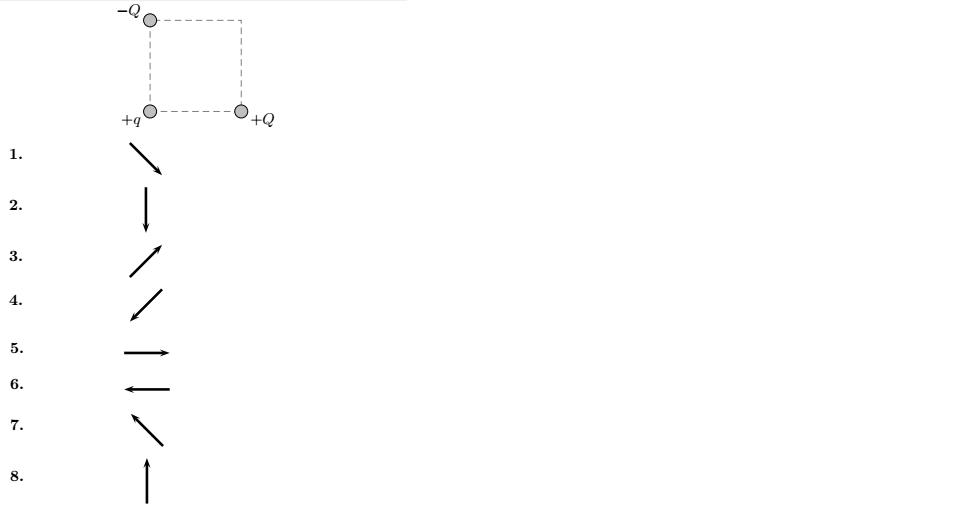Problem: Two charged particles of equal magnitude (−Q and +Q) are fixed at opposite corners of a square that lies in a plane (see figure below). A test charge +q is placed at a third corner. What is the direction of the force on the test charge due to the two other charges?

FREE Expert Solution
84% (200 ratings)
Problem Details

Two charged particles of equal magnitude (−Q and +Q) are fixed at opposite corners of a square that lies in a plane (see figure below). A test charge +q is placed at a third corner. What is the direction of the force on the test charge due to the two other charges?What scientific concept do you need to know in order to solve this problem?

Our tutors have indicated that to solve this problem you will need to apply the Coulomb's Law (Electric Force) concept. You can view video lessons to learn Coulomb's Law (Electric Force) Or if you need more Coulomb's Law (Electric Force) practice, you can also practice Coulomb's Law (Electric Force) practice problems .

What is the difficulty of this problem?

Our tutors rated the difficulty of Two charged particles of equal magnitude (−Q and +Q) are fix... as low difficulty.

How long does this problem take to solve?

Our expert Physics tutor, Juan took 2 minutes to solve this problem. You can follow their steps in the video explanation above.

What professor is this problem relevant for?

Based on our data, we think this problem is relevant for Professor Lai's class at TEXAS.